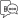Explore Categories

# Variable Related Functions

## \$\$IsSysNameVar

This function checks if the variable has a value which is a sysName like ‘Not Applicable’, ‘End of List’, etc. In case of repeated variables, if any one value is a non-sysname, it returns FALSE.

Syntax

\$\$IsSysNameVar : <Variable Specification>

Where,

<Variable Specification> is the simple variable path specification.

Example

\$\$IsSysNameVar:EmpVar

Here, \$\$IsSysNameVar returns YES if variable EmpVar has Sysname as value, else returns NO.

## \$\$IsDefaultVar

This function determines if the content of the variable has a “Default” or blank as the value. This function is applicable only for Simple variables. In case of simple repeated variable, if any one value is non-default, then this is not a default variable, and the function returns NO.

Syntax

\$\$IsDefaultVar : <Variable Specification>

Where,

<Variable Specification> is the simple variable path specification.

Example

[Field : DefaultVar]

Set as : \$\$IsDefaultVar:SVValuationMethod

\$\$IsDefaultVar returns YES if value of SV V aluationMethod is blank or Default, else returns NO.

## \$\$IsActualsVar

This function checks if the content of the variable is blank or sysname or “ACTUALS”.

Syntax

\$\$IsActualsVar : <Variable Specification>

Where,

<Variable Specification> is the simple variable path specification.

Example

\$\$IsActualsVar:SVBudget

YES is returned if the value of Variable SVBudget is Blank or Sysname or “ACTUALS”, else NO.

## \$\$IsCurrentVar

This function checks if the content of t h e variable is Blank or Sysname or “ Stock in hand”.

Syntax

\$\$IsCurrentVar : <Variable Specification>

Where,

<Variable Specification> is the simple variable path specification.

Example

\$\$IsCurrentVar:DSPOrderCombo

YES is returned if value of DSPOrderCombo is Blank or Sysname or Stock-In-Hand, else NO.

## \$\$ExecVar

This fuction returns the value of a variable in the parent report chain.

Syntax

\$\$ExecVar : <Variable Specification>

Where,

<Variable Specification> is the simple variable path specification.

Example

\$\$ExecVar:DSPShowMonthly

Function \$\$ExecVar returns the value of the variable DSPShowMonthly from the parent report.

## \$\$FieldVar

This function returns the value of the field which is acting as a variable with the specified name.

Syntax

\$\$FieldVar : <Variable Specification>

Where,

<Variable Specification> is the simple variable path specification.

Example

[Collection : GodownChildOfGodownName]

Type     : Godown

Child of : \$\$FieldVar:DSPGodownName

In this example, \$\$FieldVar is used to fetch the value of the variable DSPGodownName whose value is modified in a field. This value becomes the value for the ‘ChildOf’ attribute.

## \$\$ParentFieldVar

This function gets the field variable value from its parent report.

Syntax

\$\$ParentFieldVar : <Variable Specification>

Where,

<Variable Specification> is the simple variable path specification.

Example

[Field : ParentFieldVar]

Set as : \$\$ParentFieldVar:SVStockItem

Here, the function returns field variable value from its parent report for the variable “SVStockItem”.

## \$\$NumSets

It returns the number of columns in the report. It does not take any parameter. If the report is an auto report or sub report, it returns the number of columns in the parent of the auto/sub report. Number of set is the maximum number of values a repeated variable can hold in that report.

Syntax

\$\$NumSets

Example

[Field : CFBK Rep Col Total]

Use       : Qty Primary Field

Set as    : \$\$Total:CFBKRepParty

Border    : Thin Left

Invisible : \$\$Numsets=1

In this example, the ‘Total’ column will be invisible if there is only one column in the report.

## \$\$LowValue

This function c a n be used to get the lowest value in a set o f values in the repeated variable.

Syntax

\$\$LowValue : <Variable Specification>

Where,

<Variable Specification> is a simple variable specification.

Example

Let us suppose that the Repeat Variables in a Columnar Report are SVFromDate and SVToDate. Consider the following Field Definition in the same report:

[Field : VariableLowValue]

Use    : Name Field

Set as : \$\$LowValue:SVFromDate

\$\$Lowvalue returns the lowest value in a set of values in the repeat variable SVFromDate.

## \$\$HighValue

This function can be used to get the highest value in a set of values in the repeated variable.

Syntax

\$\$HighValue : <Variable Specification>

Where,

<Variable Specification> is a simple variable specification.

Example

Suppose that the Repeat Variables in a Columnar Report are SVFromDate and SVToDate. Consider the following Field definition in the same report:

[Field : VariableHighValue]

Use    : Name Field

Set as : \$\$HighValue:SVToDate

\$\$HighValue returns the highest value in a set of values in the repeat variable SVToDate.

## \$\$IsCommon

This function is used with repeated variable to check if all the values in the repeat set are same.

Syntax

\$\$IsCommon : <Variable Specification>

Where,

<Variable Specification> is a simple variable specification.

Example

Suppose the Repeat Variable in a columnar report is SVCurrentCompany. Consider the following Field Definition in the same report:

[Field : VariableIsCommon]

Use    : Logical Field

Set as : \$\$IsCommon:SVCurrentCompany

\$\$IsCommon returns YES if all values in SVCurrentCompany are same, otherwise returns NO.Helpful?